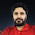## Tuesday, September 11, 2012

### Important function in x++ for AX 2009 and AX 2012

Important function in X++
I would like to share some important function in x++ Like subStr(), strCmp(),strDel() , strFind (),strfmt () , strLen (),strLwr (),strUpr (),strRep(),systemDateGet(),today(),trunc (),boxExample (),conins(), conLen(), conPeek(), conNull() which seems to easy but some time became very tough to recall in between to coding, so don’t worry and keep coding…….
Sub String in X++
// for cut a string from given posititon SubStr("ABCDEFGHIJ",7,-4) returns the text string "DEFG" (the specified sub-string).

static void subStr(Args _args)
{
str s;
real  r;
;
r = strLen("jitendraknit@gmail.com");
s = subStr("jitendraknit@gmail.com",12, 2);

print(strFmt("s=%1 and r=%2",s,r));
pause;
}

String Comparison in X++

static void strCmp(Args _args)
{
int i=2;
str s1,s2;
;
s1="string 1";
//s2="string 1";
s2="string 2";
i = strCmp(s1,s2);
if (0 == i)
{
print "s1 and s2 are the same";
}
else
{
print "s1 and s2 are different";
}
pause;
}

String Deletion in X++
static void strDel(Args _args)
{
str s;
;
s = strDel("Jitendrakumar", 5, 2);
print s;
pause;//for cut the string size first give from where it will cut thn give till thn it will cut
}

Find characters in string in X++
static void strFind(Args _args)
{
int i;
;
i = strfind("jitendrakumar", "jit", 0, 3);
if (1 == i)
{
print "Characters are found in string";
}
else
{
}
pause;
}
Strfmt()in X++
static void strfmt(Args _args)
{
str s1 ="testing";
int  s2 = 2;
real s3 =4.56;
str  s;
;
s = strfmt("string =%1,Integer = %2, Real = %3, ", s1,s2,s3);
print s;
pause;
}

Length of string in X++
// It will return no. of characters in given string
static void strLen(Args _args)
{
int i;
;
i = strLen("jitendra");
print(strFmt("i=%1",i));
pause;
}

Convert string in lower  case in X++

static void strLwr(Args _args)
{
str l;
;
l = strLwr("JITENDRA");
print(strFmt("l=%1",l));
pause;
}

Convert string in upper  case in X++
static void strUpr(Args _args)
{
str u;
;
u= strUpr("jitendra");
print(strFmt("u=%1",u));
pause;
}

Repetition of string in X++

static void strRep(Args _args)
{
str r;
;
r = strRep("xyz ", 5);
print(strFmt("r=%1",r));
pause;
}

Convert date into string in x++
// we can get date according to own format
static void systemDateGet(Args _args)
{
date d;
str d1,d2,d3 ;
;
d = systemdateget();
d1 =  date2str(d,123,2,-1,2,-1,4);
d2 =  date2str(d,231,2,-1,2,-1,2);
d3 =  date2str(d,321,2,-1,2,-1,4);

print(strFmt("dates are %1,%2,%3,%4",d,d1,d2,d3));
pause;
}

Truncate Real value in X++
// will round off all digit after decimal(.)
static void trunc(Args _args)
{
real t;
;
t = trunc(4.6789);
print strfmt("t = %1",  t);
pause;
}

static void dialogTest(Args _args)
{
Dialog dialog;
DialogGroup dialogGroup;
DialogField dialogField;
;
dialog = new Dialog("Test Dialog");
dialog.run();
}

Create box for prompt in X++

static void boxExample(Args _args)
{

if(box::yesNo("Are u sure to close this form",dialogbutton::No,"Box Title")==dialogbutton::yes)
{
print("Closing.......");
pause;
}
else
{
print("Not closing......");
pause;
}

}

Container Operation in X++
// conins to insert into container
// conLen() for the size of the container
// conPeek() to get contents of container item
// conNull() to Assign null value
static void conpeek(Args _args)
{
container c;
int i;
;
c=conNull();
c =["item1", "item2","jit"];
c= conIns(c,2,"test");
for (i = 1 ; i <= conLen(c) ; i++)
{
print conPeek(c, i);

}
pause;
}

1.nice work. it is very helpful for freshers in this technology....i appreciate!

2.3.4.5.6.